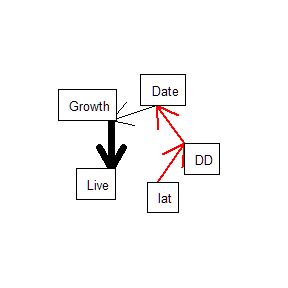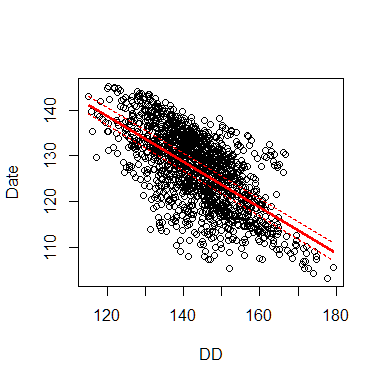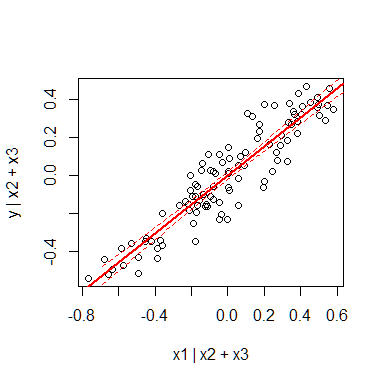# piecewiseSEM

#### 2016-12-08

The piecewiseSEM package implements piecewise structural equation modeling (SEM) for the R statistical language. SEM is useful for simultaneously testing complex multivariate hypotheses, and a piecewise approach (i.e., local estimation) is a flexible variant that allows for the fitting of various model forms and specifications.

Users are able to construct a list of structured equations corresponding to a single caustal network using the most common linear modeling functions, including: lm, rq, glm, glm.nb, gls, pgls, merMod, merModLmerTest, lme, glmmPQL, and glmmadmb.

I provide functions to evaluate (sem.fit, sem.model.fits), solve (sem.coefs), and visualize (partial.resid) the output. I also provide a way for users to translate their network to a traditional variance-covariance based SEM as implemented in the lavaan package (sem.lavaan).

In this vignette, I provide a worked example, all the way from constructing the model to conducting tests of directed separation and interpreting the results.

## Example: Shipley (2009)

The following example comes from Shipley (2009)1. The hypothetical data represent a study conducted beginning in 1970 across 20 sites differing in latitude (lat). Five individual trees are measured each year until 2006 (or until the tree dies). At each site in each year and for each individual, the following variables are measured: the cumulative degree days until bud break (Date), the Julian date of bud break (DD), the increase in stem diameter per tree (Growth) and a binary variable indicating the survival (1) or death (0) in the subsequent growing season (Live).

This dataset incorporates hierarchical observations (individual within site within year) and non-independence (repeated measures), and therefore violates some of the basic assumptions of traditional linear modeling and variance-covariance SEM, making it a perfect candidate for a piecewise approach.

The hypothesized casual structure of the network is specified by Shipley as:and will be the structure we will evaluate in this example.

# library(devtools)
# install_github("jslefche/piecewiseSEM")
library(piecewiseSEM)

### Load data from Shipley 2009

data(shipley2009)

The data is alternately hosted in Ecological Archives E090-028-S1 (DOI: 10.1890/08-1034.1).

### Create model set

We construct models corresponding to the path diagram above using a mix of the nlme and lmerTest packages, as in the supplements of Shipley (2009), and then stored in a list. glmer is used to specify a binomial distribution for survival (0 or 1). The random structure includes each observation (tree) within each site.

In each model, the response is set by the variable into which the arrow is pointing, and the predictor(s) by the variable(s) out of which the arrow(s) are originating.

This particular model specification ignores the temporal autocorrelation among years, but this could easily be incorporated as well, either in the random structure or by specifying a preset correlation structure using, for example, corAR1 or corCAR1 in the nlme package.

# Load required libraries for linear mixed effects models
library(lme4)
library(nlme)

# Load example data from package
data(shipley2009)

# Create list of models corresponding to SEM
shipley2009.modlist = list(

lme(DD ~ lat, random = ~ 1 | site / tree, na.action = na.omit,
data = shipley2009),

lme(Date ~ DD, random = ~ 1 | site / tree, na.action = na.omit,
data = shipley2009),

lme(Growth ~ Date, random = ~ 1 | site / tree, na.action = na.omit,
data = shipley2009),

glmer(Live ~ Growth + (1 | site) + (1 | tree),
family = binomial(link = "logit"), data = shipley2009)

)

### Evaluate model fit

To evaluate the fit of the SEM, we must conduct tests of directed separation. These tests essentially summarize the significance of directed relationships (unidirectional arrows in the diagram above) that could theoretically have been included in the hypothesized causal network, but which were deemed causally unimportant. In other words, are relationships left out of the causal network significant?

These tests involve fitting those missing relationships and using the resulting significance values to construct a Fisher’s C statistic. This statistic can be compared to a X distribution to assess whether the proposed causal structure represents the data, or instead whether those missing paths are causally important.

The Fisher’s C statistic can be also be used to construct a value of Akaike’s Information Criterion (AIC), to aid in the comparison of alternate structures fit to the same data.

We conduct the d-sep tests, and obtain the Fisher’s C and AIC scores, using the function sem.fit which returns a list of the following:

1. the missing paths (omitting conditional variables), the estimate, standard error, degrees of freedom, and associated p-values;
2. the Fisher’s C statistic, degrees of freedom, and p-value for the model (derived from a Chi-squared distribution);
3. the AIC, AICc (corrected for small sample size), the likelihood degrees of freedom, and the model degrees of freedom.

The argument add.vars allows you to specify a vector of additional variables whose causal independence you also wish to test. This is useful if you are comparing nested models. Default is NULL.

The argument adjust.p allows you to adjust the p-values returned by the function based on the the total degrees of freedom for the model (see supplementary material, Shipley 20132). Default is FALSE (uses the degrees of freedom reported in the summary table).

(See p-values and all that for a discussion of p-values from mixed models using the lmer package.)

sem.fit(shipley2009.modlist, shipley2009, .progressBar = FALSE)
## Conditional variables have been omitted from output table for clarity (or use argument conditional = T)
## $missing.paths ## missing.path estimate std.error df crit.value p.value ## 1 Date ~ lat + ... -0.0091 0.1135 18 -0.0798 0.9373 ## 2 Growth ~ lat + ... -0.0989 0.1107 18 -0.8929 0.3837 ## 3 Live ~ lat + ... 0.0305 0.0297 NA 1.0279 0.3040 ## 4 Growth ~ DD + ... -0.0106 0.0358 1329 -0.2967 0.7667 ## 5 Live ~ DD + ... 0.0272 0.0271 NA 1.0046 0.3151 ## 6 Live ~ Date + ... -0.0466 0.0298 NA -1.5622 0.1182 ## ##$Fisher.C
##   fisher.c df p.value
## 1    11.54 12   0.483
##
## $AIC ## AIC AICc K n ## 1 49.54 50.079 19 1431 In this case the model reproduces the data well (P = 0.484), and we reject the null that the model does not reproduce the data. The potential missing paths, including any conditional covariates, all have P-values exceeding 0.05, implying that they are not necessary to interpret the supposed causal structure of this dataset. A complementary method of assessing fit is to explore the fits of the individual component models (i.e., the structured equations). This can be done by obtaining the standard coefficient of determination (R) for each model. In the case of non-normal and mixed models, the R is calculated as a pseudo-R. More details can be found in the package documentation. sem.model.fits(shipley2009.modlist) ## Class Family Link n Marginal Conditional ## 1 lme gaussian identity 1431 0.4766448 0.6932571 ## 2 lme gaussian identity 1431 0.4083328 0.9838487 ## 3 lme gaussian identity 1431 0.1070265 0.8364736 ## 4 glmerMod binomial logit 1431 0.5589205 0.6291980 In this case, the R of the fixed effects only (marginal) ranges from 0.11 - 0.56, which is not terrible for ecological data. Acknowledging the random variation (conditional) yields improved values of R ranging from 0.62 - 0.98, implying that a large amount of variance explained can be attributed to variation among individuals and sites and not the fixed effects. ### Extract path coefficients Path coefficients can be either unstandardized (standardize = 'none', the default) or standardized (centered and scaled in units of standard deviation of the mean, standardize = 'scale', or scaled by the range the data, standardize = 'range'). The function returns a data.frame sorted by each variable and increasing significance. (coef.table = sem.coefs(shipley2009.modlist, shipley2009)) ## response predictor estimate std.error p.value ## 1 DD lat -0.8354736 0.119422385 0 *** ## 2 Date DD -0.4976475 0.004933274 0 *** ## 3 Growth Date 0.3007147 0.026631405 0 *** ## 4 Live Growth 0.3478536 0.058415948 0 *** Here we see that all relationships are significant, and that increasing latitude delays bud break. However, the later date of bud break actually enhances growth, and ultimately survival. So the interpretation of this example is that increasing latitude increases survival. We can quantify the degree to which latitude indirectly enhances survival by multiplying the path coefficients: prod(coef.table$estimate)
##  0.04349163

We can also look at the relative magnitude of the effects by scaling by mean and variance to put all coefficient estimates in units of standard deviations of the mean:

sem.coefs(shipley2009.modlist, shipley2009, standardize = "scale")
## Warning in get.scaled.data(modelList, data, standardize): One or more
## responses not modeled to a normal distribution: keeping response(s) on
## original scale!
##   response predictor   estimate   std.error p.value
## 1       DD       lat -0.7014051 0.100258794       0 ***
## 2     Date        DD -0.6281367 0.006226838       0 ***
## 3   Growth      Date  0.3824224 0.033867469       0 ***
## 4     Live    Growth  2.2268633 0.373940158       0 ***

Here, we see the strongest relationship between growth and survival, implying this is the link in the chain upon which survival mostly depends.

Its important to note that the standard errors around interactions for scaled variables (not in this example) are not valid, and therefore P-values are not reported. In every case, significance of relationships should always be assessed using the _un_standardized coefficients.

Finally, we can construct a rudimentary path diagram of the results (with variables placed equidistantly around a circle) using sem.plot:

sem.plot(shipley2009.modlist, shipley2009, standardize = "scale")
## Warning in get.scaled.data(modelList, data, standardize): One or more
## responses not modeled to a normal distribution: keeping response(s) on
## original scale!### Predictions

We can use the generic predict function to extract predicted fits based on the list of structured equations. I have slightly modified the function to return standard errors on predictions from mixed effects models using the variance of the fixed effects only (as here: GLMM Wiki - FAQ).

# Create new data for predictions
shipley2009.new = data.frame(
DD = seq(min(shipley2009$DD, na.rm = TRUE), max(shipley2009$DD, na.rm = TRUE),
by = 0.01)
)

# Generate predictions
shipley2009.new.pred = sem.predict(shipley2009.modlist, shipley2009.new)
head(shipley2009.new.pred)
##         DD Date.fit
## 1 115.0957 141.0611
## 2 115.1057 141.0561
## 3 115.1157 141.0511
## 4 115.1257 141.0461
## 5 115.1357 141.0412
## 6 115.1457 141.0362
# Plot predicted fit
with(shipley2009, plot(Date ~ DD))
lines(shipley2009.new.pred$Date.fit ~ shipley2009.new.pred$DD, lwd = 2, col = "red")

# Generate predictions with standard errors (based on fixed effects only)
shipley2009.new.pred = sem.predict(shipley2009.modlist, shipley2009.new, sefit = TRUE)

# Add 95% confidence bands (roughly 2 * SE)
lines(shipley2009.new.pred$DD, shipley2009.new.pred$Date.fit + 2 * shipley2009.new.pred$Date.se.fit, lwd = 1.5, lty = 2, col = "red") lines(shipley2009.new.pred$DD,
shipley2009.new.pred$Date.fit - 2 * shipley2009.new.pred$Date.se.fit,
lwd = 1.5, lty = 2, col = "red")### Generate variance-covariance SEM using lavaan

In cases where the model does not include non-normal distributions or random structures, the piecewise model should yield the same inferences and path coefficients as a traditional variance-covariance based SEM (where the global vcov matrix is solved simultaneously).

We can also calculate the vcov SEM using any list of model objects using the sem.lavaan function, which draws on the popular lavaan package:

(lavaan.model = sem.lavaan(shipley2009.modlist, shipley2009))
## lavaan (0.5-22) converged normally after  27 iterations
##
##                                                   Used       Total
##   Number of observations                          1431        1900
##
##   Estimator                                         ML
##   Minimum Function Test Statistic               38.433
##   Degrees of freedom                                 6
##   P-value (Chi-square)                           0.000

The output shows that the variance-covariance SEM is a worse fit (P = 0.000), indicating that a piecewise approach is justified given the hierarchical structure and non-independence of the data, both of which we have addressed through specification of a nested random structrure.

### Plot partial effect between two variables

One might be interested in the partial effects of one variable on another given covariates in the SEM. The example above is actually not a good one for this functionality, since the response in each structured equations is a direct function of a single predictor. We can, however, make up some fake data to demonstrate the utlity of calculating partial residuals.

The function we will use to evaluate the partial residuals is partial.resid and automatically returns a plot of Y ~ X | Z, where Z is any additional covariates whose effects we wish to account for.

# Create fake data
dat = data.frame(
y = runif(100),
x2 = runif(100),
x3 = runif(100)
)

dat$x1 = dat$y + runif(100, 0, 0.5)

# Create model
model = lm(y ~ x1 + x2 + x3, dat)

# Look at effect of X1 on Y given X2 and X3
partial.resid(y ~ x1, model, dat, return.data.frame = FALSE)Because we have designed this example such that x1 is only a slight variation on y, the partial residual plot after removing the effects of x2 and x3 shows a strong dependence between the two.

1. Shipley, B. (2009). Confirmatory path analysis in a generalized multilevel context. Ecology, 90(2), 363-368.

2. Shipley, B. (2013). The AIC model selection method applied to path analytic models compared using a d-separation test. Ecology, 94(3), 560-564.